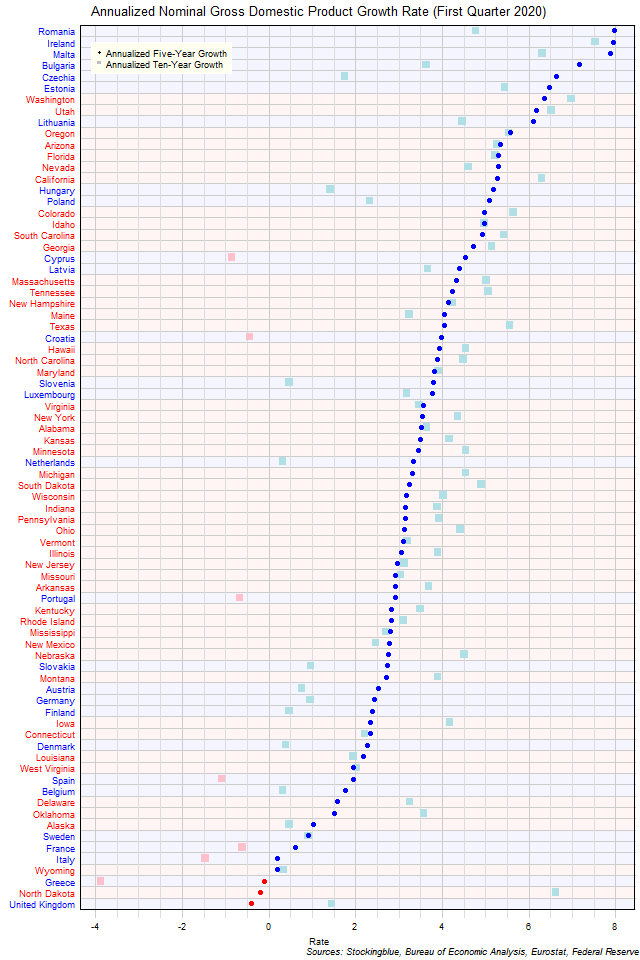# Long-Term EU and US GDP Growth Rate by State, First Quarter 2020

Sep 18, 2020The chart above shows the annualized nominal gross domestic product (GDP) growth rate when the GDP is priced in US dollars in each EU and US state over the past five years as of the first quarter of 2020 and the growth rate over the past ten years.  Only EU states saw a contraction both over the past five and ten years.

## Findings

• The difference between the state with the largest annualized five-year growth rate, Romania, and the state with the smallest, the United Kingdom, is 8.38 percentage points.
• The difference between the state with the largest annualized ten-year growth rate, Ireland, and the state with the smallest, Greece, is 11.40 percentage points.
• The median annualized five-year growth rate in the 28 EU states is 3.55% and the mean 3.73%.  The median annualized ten-year growth rate in the 28 EU states is 0.93% and the mean 1.52%.
• The median annualized five-year growth rate in the 50 US states is 3.27% and the mean 3.45%.  The median annualized ten-year growth rate in the 50 US states is 4.17% and the mean 4.10%.
• The median annualized five-year growth rate in the 78 EU and US states is 3.32% and the mean 3.55%.  The median annualized ten-year growth rate in the 78 EU and US states is 3.65% and the mean 3.18%.
• Thirty-seven states' economies grew faster over the past five years than they did over the past ten years (26 from the EU, 11 from the US).  No state had the same annualized growth rate over both periods.  The remaining 41 states saw their economies grow slower over the past five years than they did over the past ten years (2 EU, 39 US).
• All EU drops in GDP growth rate with the exception of Greece's ten-year drop are attributed to currency rate fluctuations.

## Caveats

• Data is from the first quarters of 2010, 2015, and 2020.
• The data is seasonally adjusted in current dollars.
• Euros are converted to dollars at an average exchange rate of 1.10 for the first quarter of 2020, 1.13 for the first quarter of 2015, and 1.38 for the first quarter of 2010 according to historic rates listed at the Federal Reserve (see source link below).
• US data comes in an annualized format which the EU does not, thus EU data is annualized by multiplying the quarterly figure by four.
• US growth rates may differ from those provided by the Bureau of Economic Analysis as the BEA's growth rates are based on chained dollars in conjunction with the chain index or the quality index for real GDP.  The growth rates listed here are based on nominal GDP.
• All figures are rounded to the nearest hundredth.

## Details

The United Kingdom had the worst performance over the past five years with an annualized growth rate of of -0.42%.  Romania had the best performance with an annualized gain of 7.96%.

Over the past ten years, Greece had the worst performance with an annualized growth rate of -5.87% drop in GDP while Ireland had the best performance with an annualized growth rate of 7.95%.

North Dakota had the worst change in its growth rate between its annualized ten-year growth and its annualized five-year growth slowing its rate by 6.82 percentage points.  Cyprus had the best change in its growth rate between its annualized ten-year growth and its annualized five-year growth ramping up its rate by 5.37 percentage points.

Cyprus went up 54 spots in the growth rank from having the 75th largest growth rate over the past ten years to the 21st largest growth rate over the past five years.  Conversely, North Dakota went from having the 3rd highest growth rate over the past ten years to the 77th highest growth rate over the past five years.  Only two states had annualized growth rates in the top five both over the past ten years and over the past five years: Ireland (highest ten-year, 2nd highest five-year) and Malta (5th highest ten-year, 3rd highest five-year).  Two states had growth rates in the bottom five both over both periods: Italy (77th highest ten-year, 74th highest five-year) and Greece (78th highest ten-year, 76th highest five-year).

## Sources

Federal Reserve.  2020.  "Foreign Exchange Rates."  Accessed August 21, 2020.  https://www.federalreserve.gov/releases/g5/.

US Bureau of Economic Analysis.  2020.  "GDP by State."  Accessed August 19, 2020.  https://www.bea.gov/data/gdp/gdp-state.